Refs:

## Introduction

Factor analysis is a statistical method used to describe variability among observed, correlated variables in terms of a potentially lower number of unobserved variables called factors. […] Factor analysis searches for such joint variations in response to unobserved latent(*) variables. The observed variables are modelled as linear combinations of the potential factors, plus “error” terms. The information gained about the interdependencies between observed variables can be used later to reduce the set of variables in a dataset. wikipedia.

(*) latent variables (as opposed to observable variables), are variables that are not directly observed but are rather inferred (through a mathematical model) from other variables that are observed (directly measured) wikipedia Since factor are latent we cannot use methods like regression.

So, we want to investigate if observable variables $$X_1, X_2\ldots X_N$$ are linearly related to a small number of unobservable (latent) factors $$F_1, F_2\ldots F_K$$, with $$K << N$$.

$\begin{array}{l} X_1 = w_{10} + w_{11} F_1 + \ldots w_{1K} F_K + e_1 \\ X_2 = w_{20} + w_{21} F_1 + \ldots w_{2K} F_K + e_2 \\ \ldots \\ X_N = w_{N0} + w_{N1} F_1 + \ldots w_{NK} F_K + e_N \\ \end{array}$

where $$e_i$$ are error terms, so the relation hypothesis are not exact.

The parameters $$w_{ij}$$ are called loadings.

There are the following assumptions:

• (A1) The error terms $$e_i$$ are independent from each other, $$E(e_i)=0$$ and $$var(e_i) = \sigma_i^2$$
• (A2) The unobservable factors $$F_i$$ independent from each other, $$E(F_j)=0$$ and $$var(F_j) = 1$$

(A2) is stating that these latent variables do not influence one another, which might be too strong a condition. There are more advanced models where this is relaxed (there’s an eg below).

With the loadings it is possible to compute the covariance of any two observed variables:

$Cov(X_i, X_j) = \sum_{k=1}^K w_{ik}w_{jk}$

and the variance of each $$X_i$$:

$Var(X_i) = \sum_{k=1}^K w_{ik} + \sigma_i^2$

where the sum is the communality of the variable, the variance explained by the common factors $$F_k$$. The remaining variance is called specific variance.

With all these values (if we had the loadings, which we do not), we could build a theoretical variace covariance matrix $$\Omega$$. What we have is the data, and we can use it to compute the observable variace covariance matrix $$S$$. If the model assumptions hold, then it is possible to estimate the loadings $$w_{ij}$$ of $$\Omega$$ given $$S$$. The standard way is to use principal components that uses eigenvalues and eigenvectors to bring the estimate of the total communality as close as possible to the total of the observed variances (check example 2).

Just one extra note. The values of the loadings are not unique (in fact they are infinite). This means that if the algorithm finds one solution that does not reveal the hypothesized structure of the problem, it is possible to apply a ‘rotation’ to find another set of loadings that might provide a better interpretation or more consistent with prior expectations about the dataset.

There are a number of rotations in the literatureÂ´. Some egs:

• Varimax: a rotation that seeks to maximize the variance of the squared loading for each factor (ie, make them as large as possible to capture as most signal as possible)
• Quartimax : seeks to maximize the variance of the squared loadings for each variable, and tends to produce factors with high loadings for all variables.

Rotation methods can be described as orthogonal, which do not allow the resulting factors to be correlated, and oblique, which do allow the resulting factors to be correlated.

## Factor Analysis vs. PCA

Both methods have the aim of reducing the dimensionality of a vector of random variables. Also both methods assume that the modelling subspace is linear (Kernel PCA is a more recent techniques that try dimensionality reduction in non-linear spaces).

But while Factor Analysis assumes a model (that may fit the data or not), PCA is just a data transformation and for this reason it always exists. Furthermore while Factor Analysis aims at explaining (covariances) or correlations, PCA concentrates on variances. http://www2.stat.unibo.it/montanari/Didattica/Multivariate/CA.pdf

## Example

Our sample dataset contains a hypothetical sample of 300 responses on 6 items from a survey of college students’ favorite subject matter. The items range in value from 1 to 5, which represent a scale from Strongly Dislike to Strongly Like. Our 6 items asked students to rate their liking of different college subject matter areas, including biology (BIO), geology (GEO), chemistry (CHEM), algebra (ALG), calculus (CALC), and statistics (STAT).

my.data <- read.csv("dataset_EFA.csv")
# if data as NAs, it is better to omit them:
# my.data <- na.omit(my.data)
head(my.data)
##   BIO GEO CHEM ALG CALC STAT
## 1   1   1    1   1    1    1
## 2   4   4    3   4    4    4
## 3   2   1    3   4    1    1
## 4   2   3    2   4    4    3
## 5   3   1    2   2    3    4
## 6   1   1    1   4    4    4

Package stats has a function factanal() can be used to perform factor analysis:

n.factors <- 2

fit <- factanal(my.data,
n.factors,                # number of factors to extract
scores=c("regression"),
rotation="none")

print(fit, digits=2, cutoff=.3, sort=TRUE)
##
## Call:
## factanal(x = my.data, factors = n.factors, scores = c("regression"),     rotation = "none")
##
## Uniquenesses:
##  BIO  GEO CHEM  ALG CALC STAT
## 0.25 0.37 0.25 0.37 0.05 0.71
##
##      Factor1 Factor2
## ALG   0.78
## CALC  0.97
## STAT  0.53
## BIO   0.30    0.81
## GEO           0.74
## CHEM          0.84
##
##                Factor1 Factor2
## Proportion Var    0.34    0.32
## Cumulative Var    0.34    0.66
##
## Test of the hypothesis that 2 factors are sufficient.
## The chi square statistic is 2.94 on 4 degrees of freedom.
## The p-value is 0.568
head(fit$scores) ## Factor1 Factor2 ## [1,] -1.9089514 -0.52366961 ## [2,] 0.9564370 0.89249862 ## [3,] -1.5797564 0.33784901 ## [4,] 0.7909078 -0.28205710 ## [5,] -0.1127541 -0.03603192 ## [6,] 0.6901869 -1.31361815 # plot factor 1 by factor 2 load <- fit$loadings[,1:2]
text(load,labels=names(my.data),cex=.7) # add variable names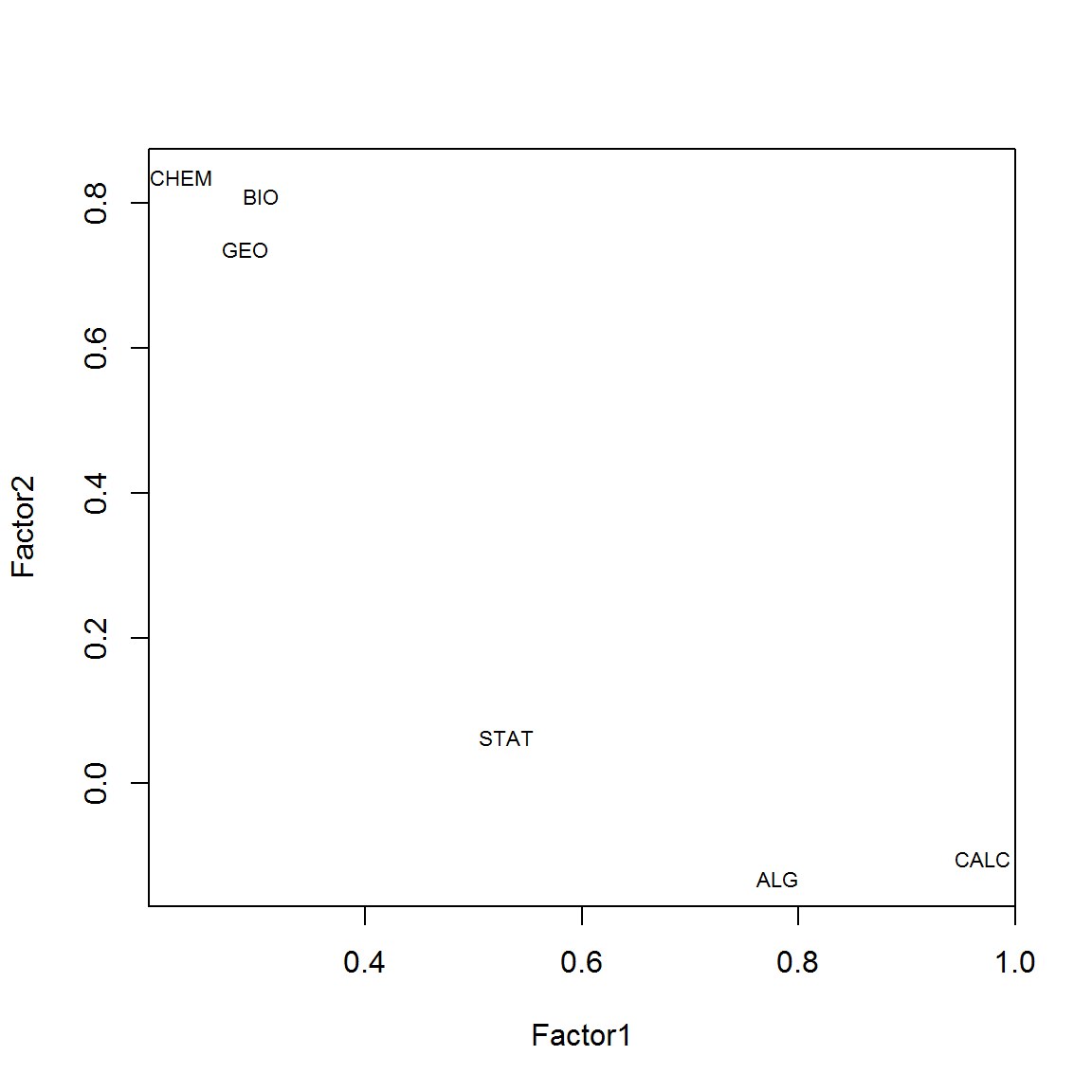The output maximizes variance for the 1st and subsequent factors, while all are orthogonal to each other.

Rotation serves to make the output more understandable, by seeking so-called “Simple Structure”: A pattern of loadings where items load most strongly on one factor, and much more weakly on the other factors. Eg, varimax rotation is an orthogonal rotation of the factor axes to maximize the variance of the squared loadings of a factor (column) on all the variables (rows) in a factor matrix, which has the effect of differentiating the original variables by extracted factor. Each factor will tend to have either large or small loadings of any particular variable. A varimax solution yields results which make it as easy as possible to identify each variable with a single factor. This is the most common rotation option. wikipedia.

fit <- factanal(my.data,
n.factors,              # number of factors to extract
rotation="varimax")     # 'varimax' is an ortho rotation

load <- fit$loadings[,1:2] load ## Factor1 Factor2 ## BIO 0.85456336 0.13257053 ## GEO 0.77932854 0.13455074 ## CHEM 0.86460737 0.05545425 ## ALG 0.03133486 0.79070534 ## CALC 0.09671653 0.97107765 ## STAT 0.16998499 0.50612151 plot(load,type="n") # set up plot text(load,labels=names(my.data),cex=.7) # add variable names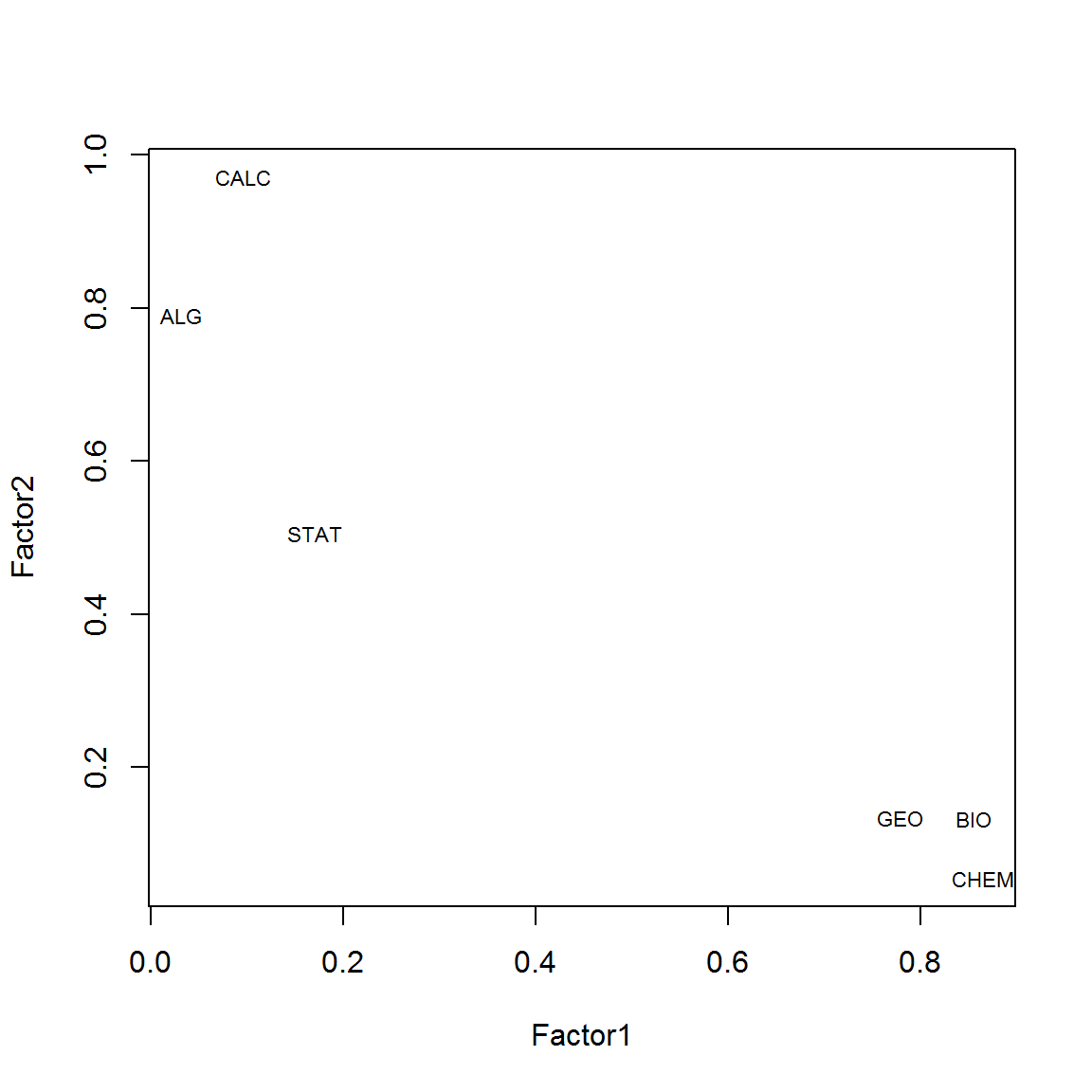Looking at both plots we see that the courses og Geology, Biology and Chemistry all have high factor loadings around 0.8 on the first factor (PA1) while Calculus, Algebra and Statistics load highly on the second factor (PA2). We could rename PA1 as Science, and PA2 as Math. Note that STAT has a much lower loading on PA2 than ALG or CALC and that it has a slight loading on factor PA1. This suggests that statistics is less related to the concept of Math than Algebra and Calculus. Just below the loadings table, we can see that each factor accounted for around 30% of the variance in responses, leading to a factor solution that accounted for 66% of the total variance in students’ subject matter preference. We could also try an oblique rotation (Stats might share some with the Science factor). Since the previous command does not implement an oblique rotation, we’ll use package psych: # install.packages("psych") # install.packages("GPArotation") library(psych) solution <- fa(r = cor(my.data), nfactors = 2, rotate = "oblimin", fm = "pa") ## Loading required namespace: GPArotation ## Warning in fac(r = r, nfactors = nfactors, n.obs = n.obs, rotate = ## rotate, : I am sorry, to do these rotations requires the GPArotation ## package to be installed plot(solution,labels=names(my.data),cex=.7, ylim=c(-.1,1))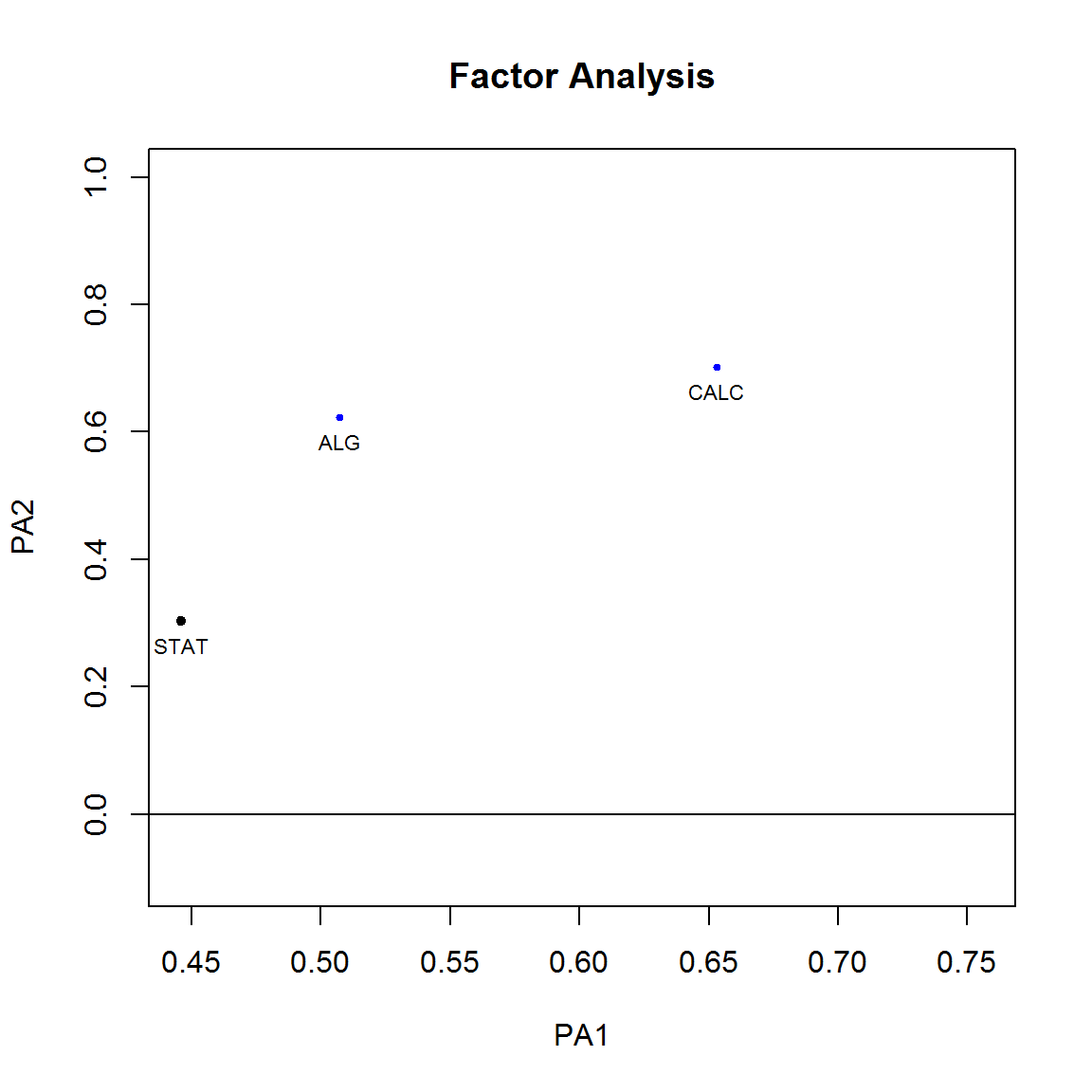solution ## Factor Analysis using method = pa ## Call: fa(r = cor(my.data), nfactors = 2, rotate = "oblimin", fm = "pa") ## Standardized loadings (pattern matrix) based upon correlation matrix ## PA1 PA2 h2 u2 com ## BIO 0.76 -0.42 0.75 0.255 1.6 ## GEO 0.71 -0.36 0.63 0.369 1.5 ## CHEM 0.72 -0.47 0.75 0.253 1.7 ## ALG 0.51 0.62 0.65 0.354 1.9 ## CALC 0.65 0.70 0.92 0.081 2.0 ## STAT 0.45 0.30 0.29 0.709 1.8 ## ## PA1 PA2 ## SS loadings 2.48 1.50 ## Proportion Var 0.41 0.25 ## Cumulative Var 0.41 0.66 ## Proportion Explained 0.62 0.38 ## Cumulative Proportion 0.62 1.00 ## ## Mean item complexity = 1.7 ## Test of the hypothesis that 2 factors are sufficient. ## ## The degrees of freedom for the null model are 15 and the objective function was 2.87 ## The degrees of freedom for the model are 4 and the objective function was 0.01 ## ## The root mean square of the residuals (RMSR) is 0.01 ## The df corrected root mean square of the residuals is 0.02 ## ## Fit based upon off diagonal values = 1 ## Measures of factor score adequacy ## PA1 PA2 ## Correlation of scores with factors 0.96 0.94 ## Multiple R square of scores with factors 0.91 0.89 ## Minimum correlation of possible factor scores 0.83 0.79 Notice that our factors are correlated at 0.21. The choice of an oblique rotation allowed for the recognition of this relationship. ## Determining the Number of Factors to Extract A crucial decision in exploratory factor analysis is how many factors to extract. This next plot is the Cattell scree plot. It plots the components/factors as the X axis and the corresponding eigenvalues as the Y-axis. As one moves to the right, toward later components, the eigenvalues drop. When the drop ceases and the curve makes an elbow toward less steep decline, Cattell’s scree test says to drop all further components after the one starting the elbow. wikipedia # install.packages("psy") library(psy) ## ## Attaching package: 'psy' ## ## The following object is masked from 'package:psych': ## ## wkappa scree.plot(fit$correlation)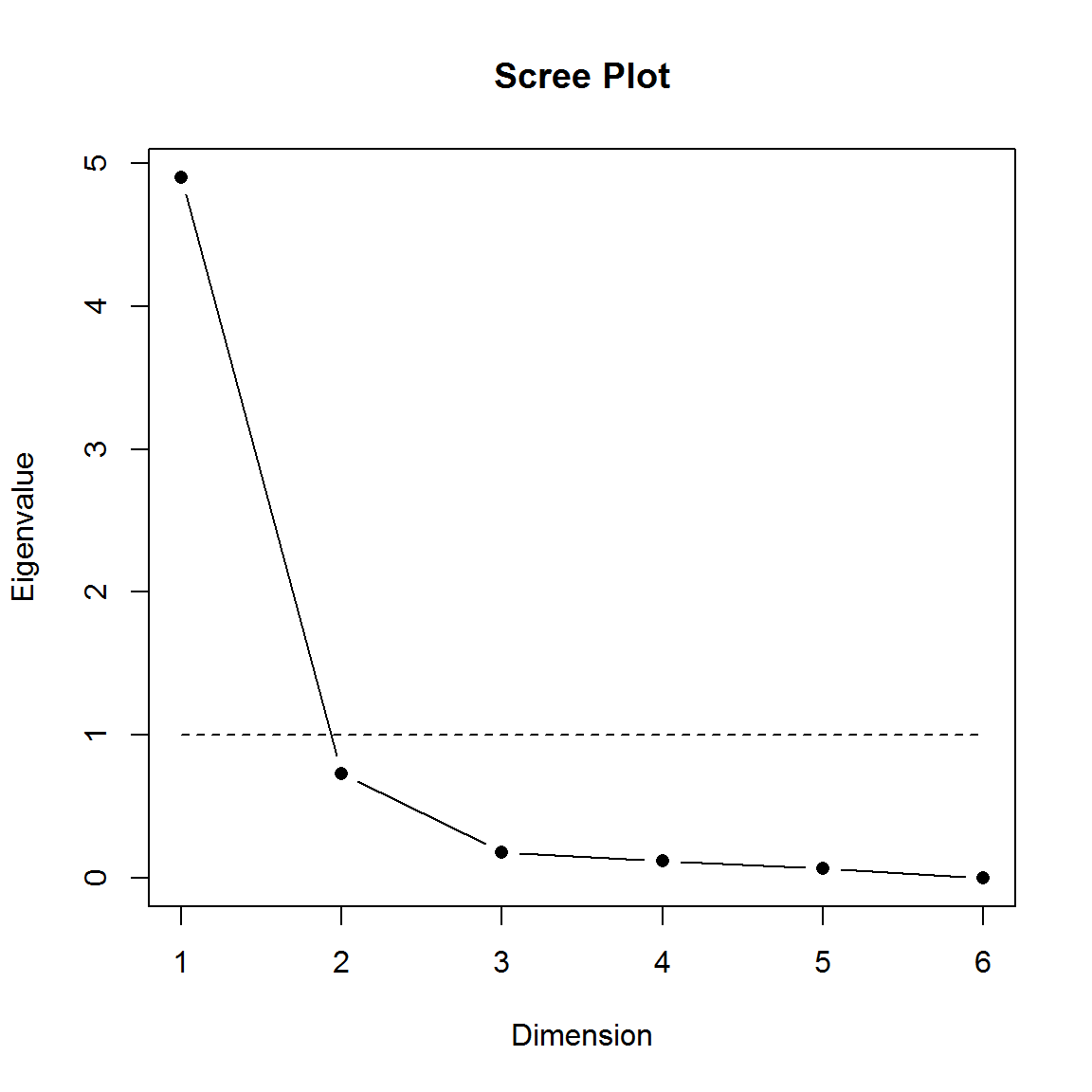Another package, nFactors, also offers a suite of functions to aid in this decision. The Kaiser-Guttman rule says that we should choose all factors with eigenvalue greater than $$1$$. More methods can be found here.

# Determine Number of Factors to Extract
# install.packages("nFactors")
library(nFactors)
## Loading required package: MASS
##
## Attaching package: 'boot'
##
## The following object is masked from 'package:psych':
##
##     logit
##
##
## Attaching package: 'lattice'
##
## The following object is masked from 'package:boot':
##
##     melanoma
##
##
## Attaching package: 'nFactors'
##
## The following object is masked from 'package:lattice':
##
##     parallel
ev <- eigen(cor(my.data)) # get eigenvalues
ap <- parallel(subject=nrow(my.data),var=ncol(my.data), rep=100, cent=.05)
nS <- nScree(x=ev$values, aparallel=ap$eigen$qevpea) plotnScree(nS)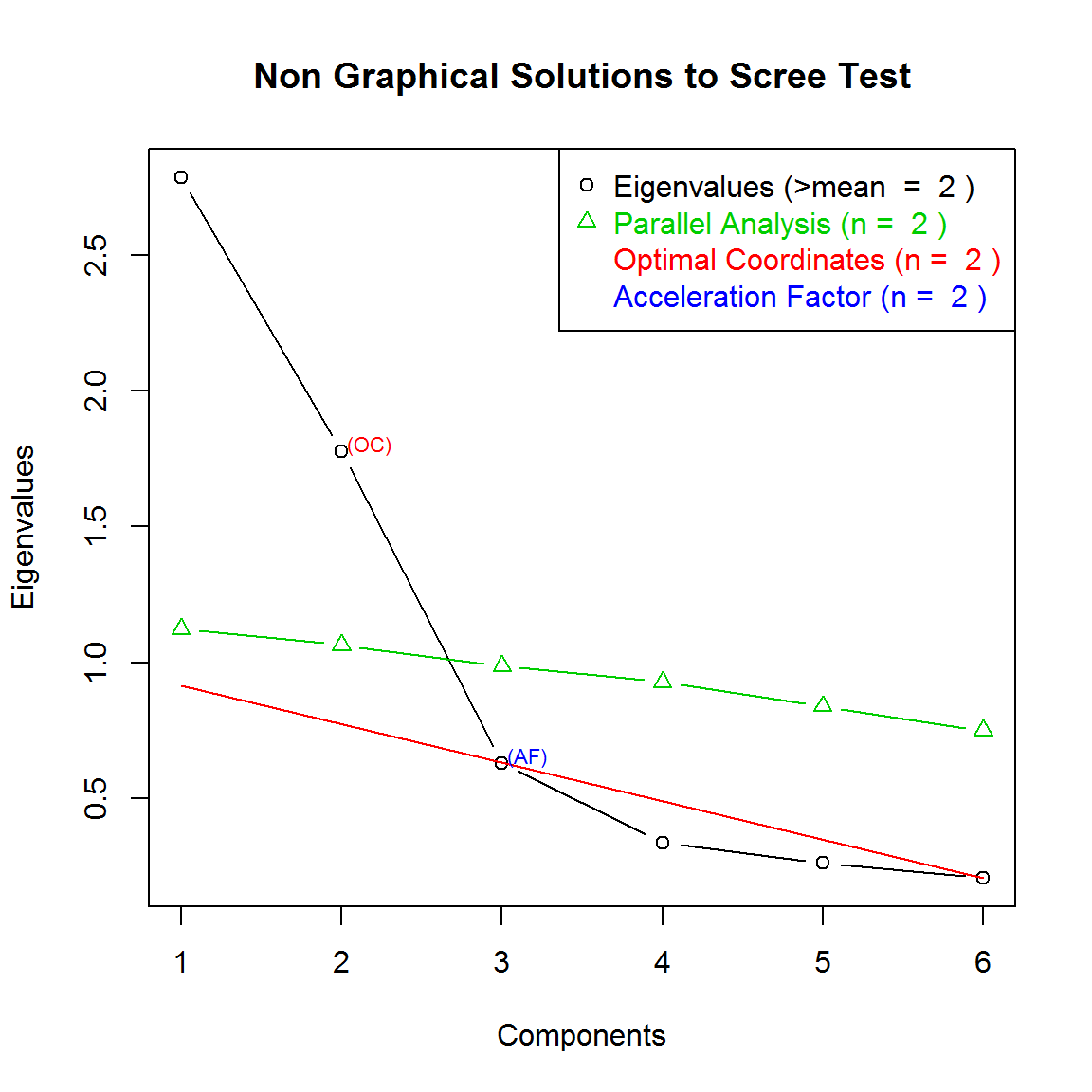## Example 2: using principal components The next file shows correlation coefficients between subject scores for a sample of 220 boys. sub_marks<-read.csv("sub_marks.csv",header=TRUE,sep=";") sub_marks  ## X Gaelic English History Arithmetic Algebra Geometry ## 1 Gaelic 1.00 0.44 0.41 0.29 0.33 0.25 ## 2 English 0.44 1.00 0.35 0.35 0.32 0.33 ## 3 History 0.41 0.35 1.00 0.16 0.19 0.18 ## 4 Arithmetic 0.29 0.35 0.16 1.00 0.59 0.47 ## 5 Algebra 0.33 0.32 0.19 0.59 1.00 0.46 ## 6 Geometry 0.25 0.33 0.18 0.47 0.46 1.00 Each subject score is positively correlated with each of the scores in the other subjects, indicating that there is a general tendency for those who do well in one subject to do well in others. The highest correlations are between the three mathematical subjects and to a slightly lesser extent, between the three humanities subjects, suggesting that there is more in common within each of these two groups than between them. In order to reduce the dimension of the problem and to explain the observed correlations through some related latent factors we fit a factor model using the principal factor method. First of all we need to compute an initial estimate of the communalities by calculating the multiple correlation coefficient of each variable with the remaining ones. We obtain it as a function of the diagonal elements of the inverse correlation matrix. R <- as.matrix(sub_marks[,-1]) icm <- solve(R) and then estimate the communalities h2.zero <- round(1 -1/(diag(icm)), 2) h2.zero  ##  0.30 0.30 0.21 0.42 0.41 0.29 Now we can compute the reduced correlation matrix by substituting the estimated communalities into the diagonal elements (the 1’s) of the original correlation matrix. R.psi <- R for (i in 1:nrow(R.psi)){ R.psi[i,i] <- h2.zero[i] } R.psi  ## Gaelic English History Arithmetic Algebra Geometry ## [1,] 0.30 0.44 0.41 0.29 0.33 0.25 ## [2,] 0.44 0.30 0.35 0.35 0.32 0.33 ## [3,] 0.41 0.35 0.21 0.16 0.19 0.18 ## [4,] 0.29 0.35 0.16 0.42 0.59 0.47 ## [5,] 0.33 0.32 0.19 0.59 0.41 0.46 ## [6,] 0.25 0.33 0.18 0.47 0.46 0.29 R.psi is still squared and symmetric, but it is not positive definite. Its decomposition through the spectral theorem shows that only two eigenvalues are positive. This means that two factors might be enough in order to explain the observed correlations. eigen(R.psi) ##$values
##   2.06689350  0.43185860 -0.07389683 -0.11987084 -0.17312229 -0.20186214
##
## $vectors ## [,1] [,2] [,3] [,4] [,5] [,6] ## [1,] -0.3894948 -0.4631310 -0.3323422 -0.08042293 0.49656626 0.5199098 ## [2,] -0.4068092 -0.3304872 0.4836944 -0.57629533 0.02029114 -0.3984924 ## [3,] -0.2898160 -0.5460418 -0.1179882 0.51251652 -0.56060428 -0.1642360 ## [4,] -0.4659483 0.4148356 -0.1362116 -0.32995348 -0.55776080 0.4150706 ## [5,] -0.4674485 0.3627504 -0.4779927 0.12767051 0.27396261 -0.5745187 ## [6,] -0.4039689 0.2728550 0.6282010 0.52304263 0.22930424 0.2038842 The estimate of the factor loading matrix will then be obtained as $$L = \Gamma L_1^{1/2}$$, where $$\Gamma$$ has in columns the first two eigenvectors and $$L_1$$ has on the diagonal the first two eigenvalues. L1 <- diag(eigen(R.psi)$values[1:2])
L1
##          [,1]      [,2]
## [1,] 2.066894 0.0000000
## [2,] 0.000000 0.4318586
Gamma <- eigen(R.psi)$vectors[,1:2] Gamma ## [,1] [,2] ## [1,] -0.3894948 -0.4631310 ## [2,] -0.4068092 -0.3304872 ## [3,] -0.2898160 -0.5460418 ## [4,] -0.4659483 0.4148356 ## [5,] -0.4674485 0.3627504 ## [6,] -0.4039689 0.2728550 We can now compute the estimated factor loadings L <- Gamma %*% sqrt(L1) rownames(L) <- names(sub_marks)[-1] # just name the rows of easier reading L ## [,1] [,2] ## Gaelic -0.5599648 -0.3043510 ## English -0.5848571 -0.2171828 ## History -0.4166596 -0.3588366 ## Arithmetic -0.6698797 0.2726131 ## Algebra -0.6720365 0.2383848 ## Geometry -0.5807737 0.1793093 The first factor seems to measure overall ability in the six subjects, while the second contrasts humanities and mathematics subjects. Communalities are, for each variable, the part of its variance that is explained by the common factors. To estimate the communalities we need to sum the square of the factor loadings for each subject: communality <- diag(L%*%t(L)) communality ## Gaelic English History Arithmetic Algebra Geometry ## 0.4061901 0.3892262 0.3023689 0.5230567 0.5084604 0.3694499 These shows, for example, that the 40% of variance in Gaelic scores is explained by the two common factors. Of course, the larger the communality the better does the variable serve as an indicator of the associated factors. To evaluate the goodness of fit of this model we can compute the residual correlation matrix $$R - L L^T$$ R - L%*%t(L) ## Gaelic English History Arithmetic Algebra ## [1,] 0.593809941 0.04640083 0.067473052 -0.002138954 0.026235892 ## [2,] 0.046400829 0.61077380 0.028380552 0.017422985 -0.021272239 ## [3,] 0.067473052 0.02838055 0.697631106 -0.021288233 -0.004469254 ## [4,] -0.002138954 0.01742299 -0.021288233 0.476943312 0.074829588 ## [5,] 0.026235892 -0.02127224 -0.004469254 0.074829588 0.491539637 ## [6,] -0.020639866 0.02927326 0.002357789 0.032069454 0.026954283 ## Geometry ## [1,] -0.020639866 ## [2,] 0.029273258 ## [3,] 0.002357789 ## [4,] 0.032069454 ## [5,] 0.026954283 ## [6,] 0.630550109 Since the elements out of the diagonal are fairly small and close to zero we can conclude that the model fits adequately the data. ## Example 3 The following correlation matrix are from 10 different intelligence tests between scores of 75 students. cor.m <- as.matrix(read.csv("qi_test.csv",header=FALSE,sep=";")) cor.m ## V1 V2 V3 V4 V5 V6 V7 V8 V9 V10 ## [1,] 1.000 0.755 0.592 0.532 0.627 0.460 0.407 0.387 0.461 0.459 ## [2,] 0.755 1.000 0.644 0.520 0.617 0.497 0.511 0.417 0.406 0.583 ## [3,] 0.592 0.644 1.000 0.388 0.529 0.449 0.436 0.428 0.412 0.602 ## [4,] 0.532 0.528 0.388 1.000 0.475 0.442 0.280 0.214 0.361 0.424 ## [5,] 0.627 0.617 0.529 0.475 1.000 0.398 0.373 0.372 0.350 0.433 ## [6,] 0.460 0.497 0.449 0.442 0.398 1.000 0.545 0.446 0.366 0.575 ## [7,] 0.407 0.511 0.436 0.280 0.373 0.545 1.000 0.542 0.308 0.590 ## [8,] 0.387 0.417 0.428 0.214 0.372 0.446 0.542 1.000 0.375 0.654 ## [9,] 0.461 0.406 0.412 0.361 0.355 0.366 0.308 0.375 1.000 0.502 ## [10,] 0.459 0.583 0.602 0.424 0.433 0.575 0.590 0.654 0.502 1.000 By looking at the correlation matrix one can see a strong correlation between the 10 tests: all the correlation values are positive and mostly varies between 0.4-0.6 Let’s factor analysis according to a maximum likelihood approach: res <- factanal(covmat=cor.m, factors=2, n.obs=75, rotation="none") res  ## ## Call: ## factanal(factors = 2, covmat = cor.m, n.obs = 75, rotation = "none") ## ## Uniquenesses: ## V1 V2 V3 V4 V5 V6 V7 V8 V9 V10 ## 0.215 0.249 0.452 0.622 0.482 0.553 0.534 0.481 0.679 0.177 ## ## Loadings: ## Factor1 Factor2 ## [1,] 0.789 -0.403 ## [2,] 0.834 -0.234 ## [3,] 0.740 ## [4,] 0.587 -0.185 ## [5,] 0.676 -0.247 ## [6,] 0.654 0.140 ## [7,] 0.641 0.235 ## [8,] 0.630 0.351 ## [9,] 0.564 ## [10,] 0.807 0.414 ## ## Factor1 Factor2 ## SS loadings 4.872 0.685 ## Proportion Var 0.487 0.069 ## Cumulative Var 0.487 0.556 ## ## Test of the hypothesis that 2 factors are sufficient. ## The chi square statistic is 16.51 on 26 degrees of freedom. ## The p-value is 0.923 Record the percentage of variability in each variable that is explained by the model (communalities): round( apply(res$loadings^2, 1, sum), 3)  # sum each row squared
##   0.785 0.751 0.548 0.378 0.518 0.447 0.466 0.519 0.321 0.823

Rotate the factors with VARIMAX. Such a rotation works on the factor loadings increasing the differences between lower weights, letting them converge to zero, and the higher weights, letting them converge to one.

res.rot<-factanal(covmat=cor.m, factors=2, n.obs=75, rotation="varimax")
res.rot 
##
## Call:
## factanal(factors = 2, covmat = cor.m, n.obs = 75, rotation = "varimax")
##
## Uniquenesses:
##    V1    V2    V3    V4    V5    V6    V7    V8    V9   V10
## 0.215 0.249 0.452 0.622 0.482 0.553 0.534 0.481 0.679 0.177
##
##       Factor1 Factor2
##  [1,] 0.852   0.245
##  [2,] 0.769   0.399
##  [3,] 0.563   0.481
##  [4,] 0.555   0.266
##  [5,] 0.662   0.281
##  [6,] 0.382   0.549
##  [7,] 0.308   0.609
##  [8,] 0.220   0.686
##  [9,] 0.375   0.424
## [10,] 0.307   0.854
##
##                Factor1 Factor2
## The p-value is 0.923